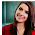# Computer System Architecture Set 14

### Questions 131 to 140

131.
Of the following phases, Identify the phase in which the programs must reside in the main memory.
 (a) Deletion (b) Printing (c) Execution (d) Reading (e) Insertion.
132.
What are the three state gates in a digital circuit?
 (a) Logic 0, Logic 1 Complement, high impedance (b) Logic 0 Complement, Logic 1, high impedance (c) Logic 0 Complement, Logic 1 Complement, high impedance (d) Logic 0, logic 1, Low impedance (e) Logic 0, logic 1, high impedance.
133.
Which of the following is false related to Stack?
 (a) Stack Pointer Points to the top most element of the stack (b) Only PUSH and POP operations are applicable (c) Implements FIFO (d) Useful for nested loops, subroutine calls etc., (e) Efficient for arithmetic expression evaluation.
134.
Which of the following is the method in which the unit receiving the data responds with another control signal?
 (a) Timing Sequence (b) Synchronous (c) Strobe (d) Short message service (e) Handshaking.
135.
Consider the following unsigned  decimal numbers
i.       6543
ii.      4444
Subtract (i) by taking the 10's complement of (ii)
 (a) 2098 (b) 2099 (c) 2096 (d) 2097 (e) 2095
136.
(x’)’ =
 (a) x2 (b) 0 (c) 1 (d) x (e) x’.
137.
Which of the following addressing modes specifies a register which contains the memory address of the operand?
138.
What is the octal equivalent of given binary number?
011001
 (a) 32 (b) 31 (c) 34 (d) 33 (e) 35
139.
Which of the following is correct related to the circuit diagrams?
 (a) Sequential circuit is an interconnection of only logic gates (b) Sequential circuit is an interconnection of only flip flops (c) Combinational circuit is an interconnection of  logic gates (d) Combinational circuit is an interconnection of flip flops (e) Part of a combinational circuit is a sequential circuit.
140.
What does D stands for in D-flip flop?
 (a) Direct (b) Don’t care (c) Double (d) Delay (e) Data.

 131 C Is the phase in which the programs must reside in the main memory. 132 E Is the three state gates in a digital circuit 133 C Stack does not Implement FIFO but implements LIFO 134 E Hand shaking is the method in which the unit receiving the data responds with another control signal 135 B 6543- 4444= 2099 136 D (x’)’ = x 137 C Register indirect addressing mode. Is the addressing mode that specifies a register which contains the memory address of the operand 138 B The octal equivalent of 011001 =31 139 C Combinational circuit is an interconnection of logic gates. 140 E D stands in D-flip flop stands for Data

29  30  31  32  33  34  35  36  37  38  39  Next >>

#### 1 comment :

1.Needed to compose you a very little word to thank you yet again regarding the nice suggestions you’ve contributed here.

Python Training in Bangalore